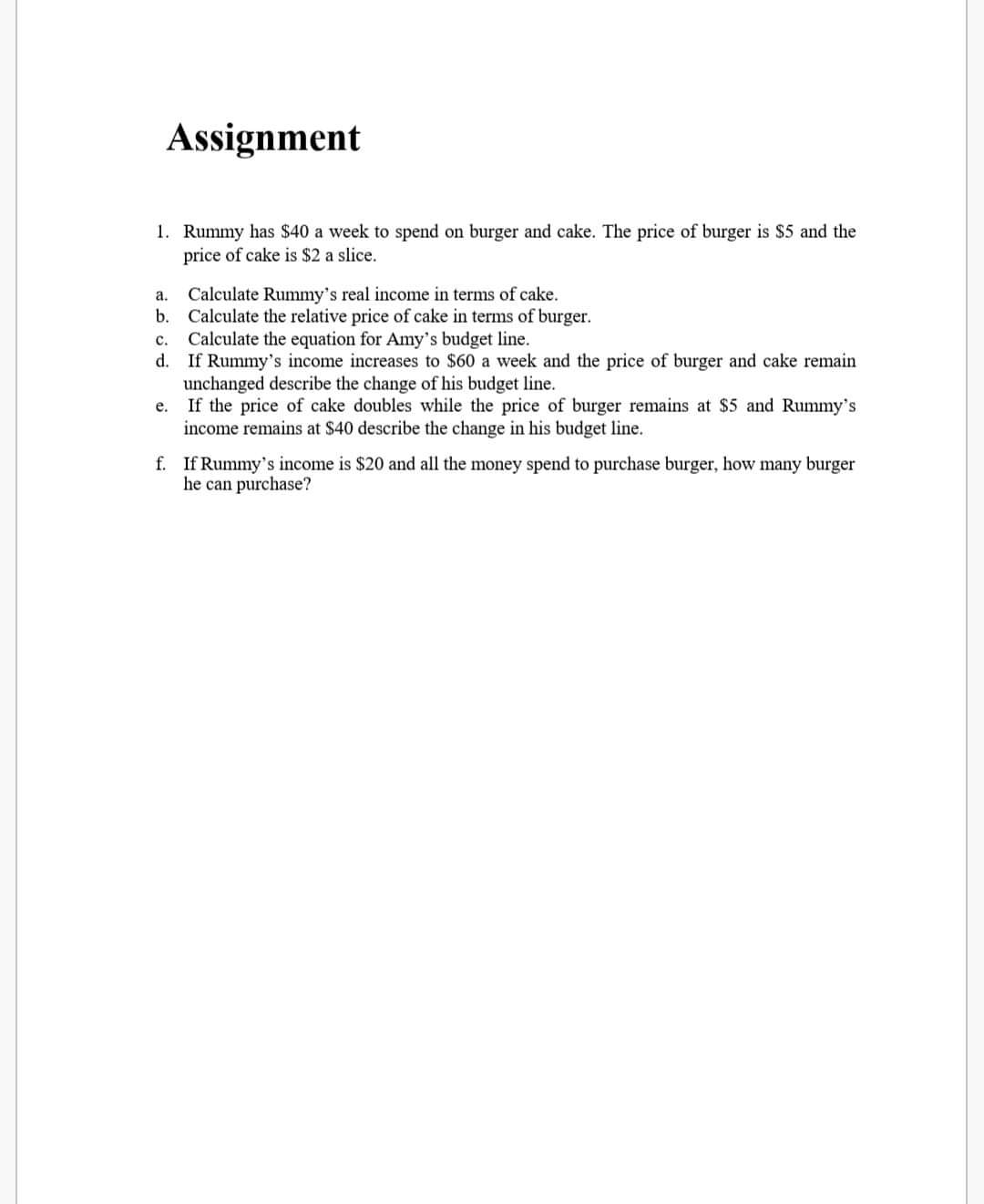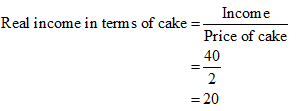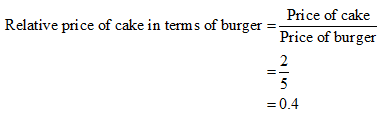# Assignment1. Rummy has \$40 a week to spend on burger and cake. The price of burger is \$5 and theprice of cake is \$2 a slice.Calculate Rummy's real income in terms of cake.b. Calculate the relative price of cake in terms of burger.c. Calculate the equation for Amy's budget line.d. If Rummy's income increases to \$60 a week and the price of burger and cake remainunchanged describe the change of his budget line.If the price of cake doubles while the price of burger remains at \$5 and Rummy'sincome remains at \$40 describe the change in his budget line.a.e.f. If Rummy's income is \$20 and all the money spend to purchase burger, how many burgerhe can purchase?

Question
195 viewshelp_outlineImage TranscriptioncloseAssignment 1. Rummy has \$40 a week to spend on burger and cake. The price of burger is \$5 and the price of cake is \$2 a slice. Calculate Rummy's real income in terms of cake. b. Calculate the relative price of cake in terms of burger. c. Calculate the equation for Amy's budget line. d. If Rummy's income increases to \$60 a week and the price of burger and cake remain unchanged describe the change of his budget line. If the price of cake doubles while the price of burger remains at \$5 and Rummy's income remains at \$40 describe the change in his budget line. a. e. f. If Rummy's income is \$20 and all the money spend to purchase burger, how many burger he can purchase? fullscreen
check_circle

Step 1

Hey, since there are multiple subpart questions posted, we will answer first three subpart question.

Step 2

a.

Rummy ‘s real income in terms of cake can be calculated as follows:Thus, the real income in terms of cake is \$20

Step 3

b.

Relative price of cake in terms of burger can be calculated as follows:Thus, the relative price of cake in terms of burger is 0.4.

...

### Want to see the full answer?

See Solution

#### Want to see this answer and more?

Solutions are written by subject experts who are available 24/7. Questions are typically answered within 1 hour.*

See Solution
*Response times may vary by subject and question.
Tagged in